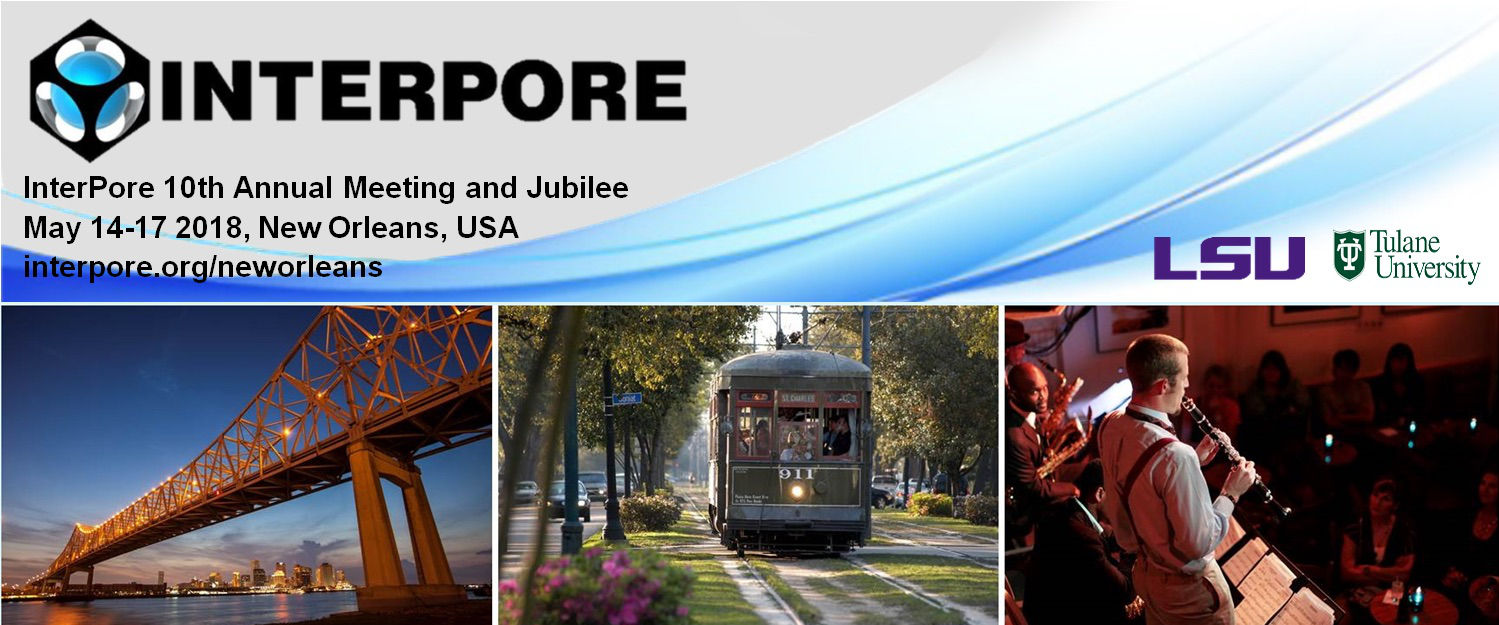#InterPore2018 New Orleans

14-17 May 2018
New Orleans
US/Central timezone

## Effects of Confinement and Surface Force on Methane Hydrate in Porous Media

15 May 2018, 15:58
15m
New Orleans

#### New Orleans

Oral 20 Minutes MS 1.12: Fluids in Nanoporous Media

### Speaker

Mr Dongliang Jin (CNRS and Laboratoire Interdisciplinaire de Physique (LIPHY), Universite Grenoble Alpes, F-38000 Grenoble, France)

### Description

Methane hydrate is a non-stoichiometric crystalline structure in which water molecules form hydrogen-bonded cages with methane molecules inside. Abundant methane hydrate resources are present on Earth, especially in various mineral porous media (e.g., clays, coal, shale, permafrost and sea-floor). Understanding the dynamics and thermodynamics of methane hydrate confined in porous media has therefore attracted a great deal of attention in the last decade.

In the present work, we combine computer modeling and theoretical approaches to determine the dynamics and thermodynamics of methane hydrate confined in porous media. First, molecular simulation, including Molecular Dynamics (MD), Monte Carlo simulations (using direct coexistence method), and free energy calculations (involving Einstein Molecule approach and umbrella sampling), is used to show that the shift in melting temperature of methane hydrate confined in porous media with respect to the bulk phase at a given pressure, $\Delta T_m = T_{m}^{pore} - T_{m}^{bulk}$, is negative. Then, the surface tension of methane hydrate $\gamma_{HS}$ and liquid water $\gamma_{WS}$ in porous media is determined to rationalize these results. We also demonstrate that the pore size ($D_p$) effect on $\Delta T_m$ can be well predicted by the Gibbs-Thompson equation, $\Delta T_m/T_m^{bulk} \sim 1/D_p$, with $\gamma_{HS} < \gamma_{WS}$. Finally, isobaric-isothermal and canonical ensemble MD simulations are used to determine several important thermodynamic properties of methane hydrate confined in porous media: (a) the thermal conductivity $k$ is evaluated by Green-Kubo integral of the autocorrelation function of the heat-flux vector (JACF); and (b) the thermal expansion $\alpha_P$ and isothermal compressibility $k_T$ are determined according to their definitions: $\alpha_P = \frac{1}{V}\left(\frac{\partial V}{\partial T}\right)_P$ and $k_T = -\frac{1}{V}\left(\frac{\partial V}{\partial P}\right)_T$. Comparison with data for bulk methane hydrate and experimental results will be made.

### References

 G. J. MacDonald, Annual Review of Energy, 1990, 15, 53